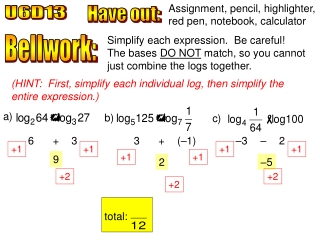DownloadDownload PresentationU6D13

# U6D13

Télécharger la présentation## U6D13

- - - - - - - - - - - - - - - - - - - - - - - - - - - E N D - - - - - - - - - - - - - - - - - - - - - - - - - - -
##### Presentation Transcript

1. Assignment, pencil, highlighter, red pen, notebook, calculator total: U6D13 Have out: Simplify each expression. Be careful! The bases DO NOT match, so you cannot just combine the logs together. Bellwork: (HINT: First, simplify each individual log, then simplify the entire expression.) a) b) c) 6 + 3 3 + (–1) –3 – 2 +1 +1 +1 +1 +1 +1 9 2 –5 +2 +2 +2

2. 1. Simplify each expression. Be careful! The bases DO NOT match, so you cannot just combine the logs together. HINT: First, simplify each individual log, then simplify the entire expression. a) b) c) 5 + 4 4 + (–1) 3 – 3 9 3 0 d) e) f) 0 – 9(1) 7(2) + (–6) –3 – (–2) –9 14 – 6 –3 + 2 8 –1

3. 1. Simplify each expression. Be careful! The bases DO NOT match, so you cannot just combine the logs together. HINT: First, simplify each individual log, then simplify the entire expression. g) h) i) 5(2) + 2(5) 5(–3) – 6(–4) 4(½) + 5(–7) 10 + 10 –15 + 24 2 – 35 20 9 –33

4. We have thoroughly covered all log topics in Algebra 2. However, there is one more thing we need to discuss that you will see on the California Standards Test (CST) in spring. Recall: you are NOT allowed any calculators on the CST. The following is a released test question: If log 2 ≈ 0.301 and log 3 ≈ 0.477, what is the approximate value of log 72?(you cannot use a calculator) log 72 Break log 72 apart into smaller factors. A 0.051 log (8 • 9) B 0.778 log (23• 32) Use the log properties to rewrite it as separate logs. C 0.861 3 log (2) + 2 log (3) D 1.857 3(0.301) + 2 (0.477) Substitute. 0.903 + 0.954 1.857

5. 2. Assume that log 4≈0.6021, log 5≈0.6990, and log 6≈0.7782. Use the properties of logarithms to evaluate each expression. DO NOT use your calculators. a) log 24 b) log 30 c) log 36 log (4 • 6) log (5 • 6) log (62) log 4 + log 6 log 5 + log 6 2 log 6 0.6021 + 0.7782 0.6990 + 0.7782 2(0.7782) 1.3803 1.4772 1.5564 d) log 125 e) log 64 f) log log (53) log (43) log (4–1) 3 log 5 3 log 4 –1 log 4 3(0.6990) 3(0.6021) –1 (0.6021) 2.0970 1.8063 –0.6021

6. 2. Assume that log 4≈0.6021, log 5≈0.6990, and log 6≈0.7782. Use the properties of logarithms to evaluate each expression. DO NOT use your calculators. g) log h) log i) log log 36 – log 5 log 4 – log 5 log (5–2) log 62 – log 5 0.6021 – 0.6990 –2 log 5 2 log 6 – log 5 –0.0969 –2 (0.6990) 2(0.7782) – 0.6990 –1.3980 0.8574

7. You know the drill it’s…. Quiz Time Clear off your desks except for a pencil, highlighter, and a ruler. No calculators! Continue working on the review when you are finished with the quiz.

8. 3. Solve for x. a) b) (exact) (approx)

9. 3. Solve for x. d) c) check! check!

10. 3. Solve for x. f) e) (exact) (approx)

11. 3. Solve for x. h) g) (exact) (exact) (approx) (approx)

12. 4. Solve each problem. a) If Yolanda deposits \$2000 in an account paying 3.8% annual interest compounded quarterly, when will her investment quadruple in value? 4 = 1.00954t log(4) = log(1.0095)4t A0 = 2000 log(4) = 4t log(1.0095) r = 0.038 4 log(1.0095) 4 log(1.0095) n = 4 A(t) = 8,000 t ≈ 36.65 years She will have \$8000 in about 36.65 years. 8000 = 2000(1.0095)4t 2000 2000

13. 4. Solve each problem. b) Roberto bought a used Honda for \$20,000. It is expected to depreciate at a rate of 16% per year. When will the car have a value of \$500. A(t) = A0(m)t 100% 500 = 20000(0.84)t – 16% 20000 20000 84% t ≈ 21.16 years 0.025 = (0.84)t m = 0.84 log(0.025) = log(0.84)t The car will be worth \$500 in 21.16 years. log(0.025) = t log(0.84) log(0.84) log(0.84)

14. Today is a workday. Complete the worksheet. The study team test is tomorrow.